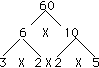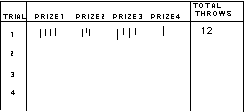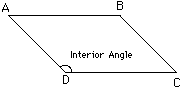Math Central - mathcentral.uregina.caResource Room— glossaries Resource RoomMathematics Glossary - Middle Years Maxine Stinka, Saskatchewan Education, Curriculum and Instruction Note: The definitions included here are those that are used in the Saskatchewan Education document "Mathematics 6-9: A Curriculum Guide for the Middle Level". Various mathematics dictionaries may have different definitions. These definitions are designed to be meaningful to middle level mathematics teachers. Select the first letter of the term that you wish to look for. A | B | C | D | E | F | H | I | J | K | L | M | N | O | P | Q | R | S | T | U | V | W | X | Y | Z F face any of the flat sides of a polyhedron. factor one of the numbers that make up a number by multiplication. E.g. 3 is a factor of 6 since 6 = 3x2.. factor tree a diagram representing a systematic way of determining all the prime factors of a number. e.g.flip see reflection. fraction see rational number any number that can be written in the form a/b where a and b are integers and b cannot equal 0. It is a negative fraction number if it is less than zero i.e. either a or b, but not both, is negative. frequency diagram used in statistics as a method of recording the data collected. A tally is often used in the frequency diagram to keep track of the number of times something occurs.frustum the region a solid cone or pyramid between the base and a plane parallel to the base.function a set of ordered pairs where each first element is paired with one and only one second element and no element in either pair is without a partner. G golden rectangle a rectangle where the ratio of its length to its width is (1 + sqrt 5)/2 :1, i.e. about 1.618:1. H hectare (hm2) a unit of area that is 100 m by 100 m. It is equivalent to 10 000 m2. height of a triangle see altitude. histogram a type of statistical graph that uses bars, where each bar represents a range of values and the data is continuous.   hypotenuse the side opposite the right angle in a right triangle. It is always the longest side in the triangle. I   image the result of a transformation on an object. improper fraction a fraction whose numerator is greater than or equal to its denominator. e.g. 11/7. Note: 11/7 and 1 4/7 are both in simplest form. inequality a mathematical sentence that includes one of the symbols >, <, or the symbols for greater than or equal to, less than or equal to, or not equal. integer any number in the set 0,+/- l,+/- 2,+/- 3, ... interior angles of a polygon angles within a polygon formed by the intersection of two sides.interest money paid for the use of someone else's money. Simple interest is calculated using the formula i = prt. Compound interest is calculated by adding the interest on to the principal each time the interest is calculated. isosceles a polygon with two sides equal in length. Usually used to refer to either a triangle or a trapezoid. Questions or comments about the glossary can be directed to TheCentralizer@MathCentral.uregina.ca or see About Us page for more information.
 Math Central is supported by the University of Regina and the Imperial Oil Foundation.about math central :: site map :: links :: notre site français* Registered trade mark of Imperial Oil Limited. Used under license.